View Course Path

# Digital Number Systems And Base Conversions

Hello. Namaste. Bonjour. Ola. Salam. Hallo.

Same word. But represented in different forms/ languages.

There are about 6500 languages in the world today. When we travel to a new country, we try adapting to their culture by first inculcating their language and learning their lifestyle. We know what we want to convey to them, but just the words need to be learned. Imagine. A simple word. We can say a simple word in 6500 different ways. With such diverse dialects among humans, don’t you wonder if there is something similar used in the digital world too? Do machines and software have different languages to convey various information? Is there more than one language? Is there a translation possible for these languages? And can we, as humans, understand these translations as well?

`Computers describe the number system in many different forms and symbols. We have:

1. The Binary Number System
2. The Decimal Number System
3. The Octal Number System and

In this article, we shall go through each of these systems and learn what they are, where do we see its applications and the conversion mechanism between these systems.

Contents

## What is the Binary Number System?

We often come across the word ‘binary’ in mathematics and digital electronics. But what exactly is this system?

The word ‘binary’ comes from the Latin word binarius, which means ‘duality’ or ‘a pair.’ In simple English, bi- also means two. Thus, the Binary Number System consists of only two values – a 0 and a 1. Every real number can be written in 0s and 1s as the sum of powers of 2. This number system is also known as a base-2 number system.

In digital electronics, we represent information in the form of bits, which is the smallest unit in the digital technology. The word ‘bit’ comes from ‘Binary digIT.’

Then again, if we only have two values, that is 0 and 1, how do we illustrate the entire number system? How do we represent, maybe, 4, 11, 16, or 1996?

### Representation of values with the Binary Number System

In the Binary Number System, a 0 is represented with a 0, and a 1 is described with 1. We attach a subscript 2 to the number, written (x)2 to specify that we are implementing the Binary Number System. But how to give an accurate representation?

Start back at 0 and add a 1 to the left. 10. This is the number 2 in the Binary Number System. Next, begin with 1 and add a 1 to the left. 11. This is the number 3.

For the number 4, begin with a 0 first. Adding a 1 next would give us the number 2. So add a 0. But this brings us to the original number 0. So, add 1 to the left again. 100. This is number 4.

 NUMBER BINARY STEPS TO REPRESENT 0 0 Represented with a 0 1 1 Represented with a 1 2 10 Begin with a 0 again, but add 1 on its left 3 11 Begin with a 1 again, but add 1 on its left 4 100 Place a 0 again, and place 1 to the number on the left. But the number 10 already exists. Thus, 1 is added to the next position on the left 5 101 … 6 110 … 7 111 … 8 1000 Begin again with 0 at three places. Then, add 1 on the left. 9 1001 Continue with the same!

Thus, we can continue this step for writing down numbers.  But is this a feasible method for writing down large numbers like 3000? Can we continue adding 0’s and 1’s one by one until we get the final value for the number 3000?

In spite of having this method, there is another way of representing values in the Binary Number System.

Let X be either 0 or 1. We shall learn this method by considering the number 11 as an example.

Step 1: Breakdown the number 11 as a sum of the exponential multiple of 2 less than/equal to 11. Thus, 11 can be represented a sum of 1, 2 and 8 (1+2+8 = 11)

Step 2: Represent these values in the base-2 number system. Thus, 1 is represented as 20, 2 is represented as 21, and 8 is represented as 23.

Step 3: Consider the image above. Fill in the value 1 in place of X for 20, 21, and 23. The remaining boxes can be filled with 0s.

Thus, (1011)2 is the Binary representation of the number 11.

Easy? Or confused? Let us repeat this process for another number. Consider the number 16.

Step 1: Breakdown the number 11 as a sum of the exponential multiple of 2 less than/equal to 11. Thus, 16 can be simply be represented a sum of 16 itself as it is the most significant exponential multiple of 2 corresponding to its value.

Step 2: Represent these values in the base-2 number system. Thus, we write 16 as 24.

Step 3: Consider the same image as used previously. Fill in the value 1 in place of X for 24. Fill the remaining boxes with 0s.

(10000)2 is the binary representation of the number 16.

Easy-peasy right? Rather than wasting time on the previous method, this method is more quicker, relevant, and simpler to use.

Why not try out a few numbers yourself? Here is Question 1 for you! Try converting the numbers 22, 7, and 71 and leave your answers in the comments!

## Where do we use the Binary Number System?

The applications of the Binary Number System can be found vastly in computer technology. Almost every language and programming uses the base-2 number system for digital encoding. Microcontrollers and microcontrollers use only binary logic in computing. Also, the Binary Number System is also found in the mathematical concept of Boolean Algebra.

## What is the Decimal Number System?

The Decimal Number System is pretty much the same as the real number system. Every real number is written using ten digits – 0, 1, 2, 3, 4, 5, 6, 7, 8, and 9, as the sum of powers of 10. This system is also called a base-10 system. To depict a number in the Decimal Number System, we attach a subscript 10 to x, written x10.

When we write down a number in this system, the rightmost bit is called the Least Significant Bit (LSB), and the leftmost bit is the Most Significant Bit (MSB).

### Representation of values with the Binary Number System

The Decimal Number System has numbers 0-9. Just like the real number system. But what comes next?

It is simple. After the number 9, place a 0. To the left of the zero, add a 1. We get 10. Next, keep the 1 in the tens position as it is, and update the remaining values from 1-9. Thus, we get 10, 11, 12….19. After 9, again place a 0 at the one’s place. Now, place a 2 to the left and continue the same procedure as done previously. This is pretty much the same as the real number system itself.

Let X be any number between 0 to 9. We shall learn this method by considering the number 2125 as an example.

Step 1: Breakdown the number 2025 as a sum of the ones, tens, hundreds, thousands, and so on. Thus, we can write 2025 as a sum of 2000, 100, 20 and 5 (2000+100+20+5=2025)

Step 2: Break down each of these numbers as a product of an exponential multiple of 10 and a number between 0-9. (2000 = 2 x 1000, 100 = 1 x 100, 20 = 2 x 10, 5 = 5 x 1)

Step 3: Represent these values in the base-10 number system. Hence, we have 2000 = 2 x 103, 100 = 1 x 102, 20 = 2 x 101 and 5 = 5 x 100

Step 4: Consider the image above. Fill in the value the values of 0-9 as derived in the previous step, in place of X for 100, 101, 102, and 103. Fill in the remaining boxes with 0s.

Thus, the decimal representation of the given number is (2125)10. The LSB is 5, and the MSB is 2 in this case.

Let us test your understanding of this concept. Question 2 here! Guess the LSB and MSB of the given numbers – 0411, 2210, and 49.

## Where do we use the Decimal Number System?

We use this system for all our arithmetic operations in our daily life. Since it is easily readable by humans, it is widely used, as compared to the other systems.

## What is the Hexadecimal Number System?

Imagine writing the binary conversion of the number 9876543210. It would take so much time to determine the sum of powers of 2, and the resulting base-2 number would be vast. When the computers and microcontrollers need to read this value, it would take so much time in reading this number, in understanding the value, and then performing operations on it. It would be more convenient to have some system which can represent the number more shortly, as well as makes it easier to understand.

We can do this by grouping the entire binary converted value in groups of 4, with each group being represented with a newer variable. Thus, this new system of groups of 4 bits uses a system called the Hexadecimal Number System.

Thus, the ‘Hex’ numbering system is a base-16 system and is the widely used system for representing large number systems.

### Representation of values with the Hexadecimal Number System

We have already said that in the Hexadecimal Number System, we form groups of 4 binary values as one single Hex value. When we take the place value of each of these places for the largest possible 4digit binary number (1111)2 (8+4+2+1), we get 15. This covers up to 16 values starting from 0, and hence we represent any real number as a combination of 16 different values. To specify that it is represented using this system, we attach a subscript 16 to the converted number, written (x)16. But how to go ahead with this?

Since there are 16 digits which can represent a number, do we start representing them from 0,1,2,3,… all the way to 13,14,15? Would it not be confusing between ‘12’ and ‘1’’2’ together? Is there a more straightforward way of resolving this?

Among the 16 values, we start from 0,1,2… till 9. But after 9, instead of continuing with 10, we begin with A., So, the remaining values are A, B, C, D, E, and F. Confused seeing alphabets in a ‘number representation’ system? Don’t worry! We shall simplify it for you.

 NUMBER BINARY REPRESENTATION HEXADECIMAL VALUE 0 0000 0000 0 1 0000 0001 1 2 0000 0010 2 3 0000 0011 3 4 0000 0100 4 5 0000 0101 5 6 0000 0110 6 7 0000 0111 7 8 0000 1000 8 9 0000 1001 9 10 0000 1010 A 11 0000 1011 B 12 0000 1100 C 13 0000 1101 D 14 0000 1110 E 15 0000 1111 F

We have listed the representation of numbers up to 15. How do we move on with 16?

Step 1: Given a real number, represent it in its binary equivalent. Thus, 16 can be represented as (10000)2

Step 2: We must now form groups of 4 each. Since 5 bits are present above, we add 3 additional 0 bits (this does not change any value). Thus, 16 is now written as (00010000)2. For convenience, we shall separate the entire 8 bit as (0001 0000)2.Step 3: Now, take each of these 4-bit groups, and convert them to their equivalent Hexadecimal values. Thus, (0001) is 1 and (0000) is 0 in the Hex system.

Step 4: Combine these values together. (10)16 is thus, the Hexadecimal equivalent of the real number 16. We can also represent it as (1x16)+0 = (10)16

A little different from the previous methods. But it is easy, now that we know how it works, isn’t it? This conversion needs a little time to be understood, but once you get a hang on the method, it is easy peasy lemon squeezy!

You might have a little doubt in this method still. Why not try testing your level of understanding!? Question 3 requires you to convert the real number 72 and 25 to their Hex equivalents. Leave the answers with the question numbers in the comments below!

## Where do we use the Hexadecimal Number System?

The Hex system can be used in defining memory locations in the computer. These memory locations are required to display error messages in programming as well. We also use them to describe the colours on the colour palette for webpages. This is done with 6 Hex digits, with the first 2 Hex digits representing the amount of red, next two representing the presence of green, and the last 2 digits showing the amount of blue present in the actual colour. #FFFFFF is White Colour, and #000000 is Black Colour Code. The Hex System is also used to represent Media Access Control (MAC) addresses in networking.

## What is the Octal Number System?

Octagon. Octopus. The prefix oct- is common in both cases. But what does it have to do with the Octal Number System?

Octagon is a polygon with 8-sides, and Octopus is a marine being with 8 legs. This suggests that the OCTAL Number System might be linked around the number 8 as well!

The Octal Number System, also called the Oct system, is another number representation system commonly used. This system is also called the base-8 system. Thus, we can represent 8 values in this system, that is 0,1,2,3,4,5,6,and 7.

This system is also used in shortening large binary values to smaller convenient forms to make is easily understandable. The principle is pretty much similar to that of the Hex system. Where we collected bits of the Binary Number system in groups of 4 for the Hex system, we now gather the bits in groups of 3 for the Oct System. Let us see how exactly this is used.

### Representation of values with the Octal Number System

We have already discussed that in the Octal Number System, we form groups of 3 binary values as one single Oct value. When we take the place value of each of these places for the largest possible 3 digit binary number (111)2 (4+2+1), we get 7. This covers up to 8 values from 0 to 7, and hence we represent any real number as a combination of 8 different values. To specify that it is represented using this system, we attach a subscript 8 to the converted number, written (x)8. But how to go ahead with this?

 NUMBER BINARY REPRESENTATION OCTAL VALUE 0 000 0 1 001 1 2 010 2 3 011 3 4 100 4 5 101 5 6 110 6 7 111 7

We have listed down the values from 0 to 7, but how to show 8 in the Octal system?

Step 1: Given the number 8, represent it in its binary equivalent. Thus, 8 can be represented as (1000)2

Step 2 : We must now form groups of 3 bits each. Since 4 bits are present above, we add 2 additional 0 bits (this does not change the value). Thus, 8 is now written as (001000)2. For convenience, we shall separate the entire 6 bits as (001 000)2.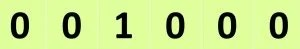Step 3 : Now, take each of these 3 bit groups, and convert them to their equivalent Octal values. Thus, (001) is 1 and (000) is 0 in the Oct system.

Step 4 : Combine these values together. (10)8 is thus, the Octal converted equivalent of the real number 8. Also, we can simply break down 8 as (1x8)+0=(10)8

Really similar to the previous system! Thus, if we know either of the Hexadecimal or Octal system, the other system is pretty much same to deal with!

Question time! Convert the numbers 100 and 50 in the Octal system and mention the answers of this Question 4 in the comments!

## Where do we use the Octal Number System?

On UNIX, Linux, and Mac OS systems, it is used to represent file permissions. We also use this system in representing UTF8 numbers. Sometimes, it is used within digital displays for clocks, timers, elevators, etc.

## Binary to Decimal Conversion Method

This conversion might be very easy for you, as we have seen the representation of Binary Number System. Nevertheless, we shall see how this works out.

For example, we shall convert the binary number (101010)2 to its equivalent Decimal number.

Step 1: Assign the place values for each digit in the sequence, starting from 20 for the LSB, and increase the exponential value while moving towards the left, by value of one. Thus, we get the following substitution.Step 2: Now, multiply these exponential powers with each of their respective binary bit values, and add all the products.Step 3 : The sum derived is the equivalent Denary value. Thus, (1×25)+(0x24)+ (1×23)+(0x22)+ (1×21)+(0x20) gives a sum of (42)10.

## Decimal to Binary Conversion Method

We have already seen this previously. Let us see another example for this method.

Consider the number 16.

Step 1: Breakdown the number 16 as a sum of the exponential multiple of 2 less than/equal to 11. Thus, 16 can be simply be represented a 16 itself as it is the largest exponential multiple of 2 equal to its value.

Step 2: Represent these values in the base-2 number system. Thus, 16 is represented as 24.

Step 3: Consider the same image as used previously. Fill in the value 1 in place of X for 24. The remaining boxes can be filled with 0s.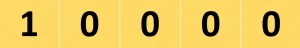(10000)2 is the binary representation of the number 16.

## Binary to Octal Conversion Method

We shall portray this conversion with (1101)2 as an example.

Step 1 : Octal conversions require us to group the bits in groups of 3. Since only 4 bits are present, add 2 more 0s to make 2 groups of 3 bits each. For convenience, we shall separate the entire 6 bits as (001 101)2.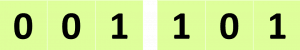Step 2 : Now, take each of these 3 bit groups, and convert them to their equivalent Octal values. Thus, (001) is 1 and (101) is 5 in the Oct system.

Step 3 : Combine these values together. (15)8 is thus, the Octal converted equivalent of (1101)2.

## Octal to Binary Conversion Method

We shall convert the Octal number (24)8 to the binary value.

Step 1 : Consider each digit of the Octal number as separate values.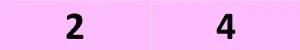Step 2: We know that each Octal digit is comprised of 3 bits. Thus, split every Octal digit equal to its binary bits.

Thus, (10100)2 is the converted binary value of (24)8.

## Octal to Decimal Conversion Method

Considering (33)8 as an example, we shall convert it into its Decimal equivalent.

Step 1 : Consider each digit of the Octal number as separate values.Step 2: We know that each Octal digit is comprised of 3 bits. Thus, split every Octal digit equal to its binary bits.Step 3: Now, assign the place values for each digit in the sequence, starting from 20 for the LSB, and increase the exponential value while moving towards the left, by value of one. Thus, we get the following substitution.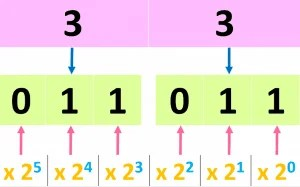Step 4: Now, multiply these exponential powers with each of their respective binary bit values, and add all the products.Step 3 : The sum derived is the equivalent Denary value. Thus, (0x25)+(1×24)+ (1×23)+(0x22)+ (1×21)+(1×20) gives a sum of (27)10.

## Decimal to Octal Conversion Method

We shall do the conversion for the number 15.

Step 1 : Given the number 15, represent it in its binary equivalent. Thus, 15 can be represented as (1111)2Step 2 : We must now form groups of 3 bits each. Since 4 bits are present above, we add 2 additional 0 bits (this does not change the value). Thus, 15 is now written as (001111)2. For convenience, we shall separate the entire 6 bits as (001 111)2.Step 3 : Now, take each of these 3 bit groups, and convert them to their equivalent Octal values. Thus, (001) is 1 and (111) is 7 in the Oct system.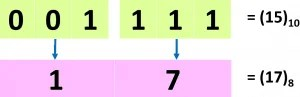Step 4 : Combine these values together. (17)8 is thus, the Octal converted equivalent of the real number 15. Also, we can simply break down 15 as (1x8)+7 =(17)8

## Hexadecimal to Decimal Conversion Method

We will convert (AB)16 to the decimal value.

Step 1 : Consider (AB)16 as two separate variables; convert each Hex value to its binary equivalent.Step 2: Now, simply convert the binary value to its decimal equivalent.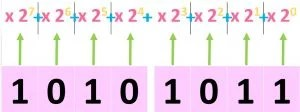Step 3: Adding the weighted values, we get (1×27)+ (1×25)+ (1×23)+ (1×21)+ (1×20) gives us (171)10.

## Decimal to Hexadecimal Conversion Method

Let us convert the number 20 to the Hex form.

Step 1 : Given a real number, represent it in its binary equivalent. Thus, 20 can be represented as (10100)2.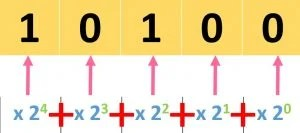Step 2 : We must now form groups of 4 each. Since 5 bits are present above, we add 3 additional 0 bits (this does not change any value). Thus, 20 is now written as (00010100)2. For convenience, we shall separate the entire 8 bit as (0001 0100)2.Step 3 : Now, take each of these 4 bit groups, and convert them to their equivalent Hexadecimal values. Thus, (0001) is 1 and (0100) is 4 in the Hex system.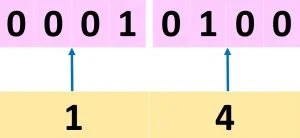Step 4 : Combine these values together. (14)16 is thus, the Hexadecimal equivalent of the real number 16. We can also represent it as (1x16)+0 = (10)16

## Binary to Hexadecimal Conversion Method

We will consider the binary value (101100)2 for the conversion.

Step 1 : Hexadecimal digits represent 4 bits each. We must now form groups of 4 bits each. Since 6 bits are present above, we add 2 additional 0 bits (this does not change the value). Thus, (101100)2 is now written as (00101100)2. For convenience, we shall separate the entire 8 bit as (0010 1100)2.Step 2 : Now, take each of these 4 bit groups, and convert them to their equivalent Hexadecimal values. Thus, (0010) is 2 and (1100) is C in the Hex system.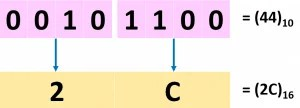Step 3 : Combine these values together. (2C)16 is thus, the Hexadecimal equivalent of (101100)2.

## Hexadecimal to Binary Conversion Method

We will convert (A1)16 to the decimal value.

Step 1 : Consider (A1)16 as two separate variables.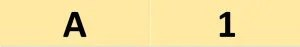Step 2: Now, convert each Hex value to its decimal equivalent.Thus, (1010001)2 is the binary equivalent of (A1)16.

In summary, we have come across the different number systems in digital electronics as well as the conversions between them.

Goodbye. Alvida. Auf Wiedersehen. Ciao. Adieu

## 6 thoughts on “Digital Number Systems And Base Conversions”

1.Hari Prasanth says:

I find this article to be resourceful while refreshing my basics. However, I find it surprising of not seeing the L division rule ( Divide by 2) to move from Octal, decimal, Hexadecimal number system to binary number system. That would be even more easy-peasy if properly understood.

1.Umair Hussaini says:

Yeah that’s a fairly easy way to convert Decimal to Binary! I think we can add that to this post. Thanks for your comment Mr. Prasanth!

2.Anvitha says:

Hi
22= 10110
7= 111
71= 1000111

1.Umair Hussaini says:

Correct!

3.Supreeth says:

This is the best article I’ve come across! Extremely easy to understand, and fun to learn too

1.Umair Hussaini says:

Awesome! Thanks for letting us know Supreeth!

This site uses Akismet to reduce spam. Learn how your comment data is processed.